×#### Thank you for registering.

One of our academic counsellors will contact you within 1 working day.

Click to Chat

1800-1023-196

+91-120-4616500

CART 0

• 0

MY CART (5)

Use Coupon: CART20 and get 20% off on all online Study Material

ITEM
DETAILS
MRP
DISCOUNT
FINAL PRICE
Total Price: Rs.

There are no items in this cart.
Continue Shopping• Complete JEE Main/Advanced Course and Test Series
• OFFERED PRICE: Rs. 15,900
• View Details

```Chapter 13: Linear Equation in Two Variables Exercise – 13.4

Question: 1

Give the geometric representations of the following equations

(a) on the number line (b) on the Cartesian plane:

(i) x = 2

(ii) y + 3 = 0

(iii) y = 3

(iv) 2x + 9 = 0

(v) 3x - 5 = 0

Solution:

(i) We are given, x = 2

The representation of the solution on the number line, when given equation is treated as an equation in one variable.The representation of the solution on the Cartesian plane, it is a line parallel to y axis passing through-the point (2, 0) is shown below(ii)  We are given, y + 3 = 0

We get, Y = – 3

The representation of the solution on the number line, when given equation is treated as an equation in one variable.The representation of the solution on the Cartesian plane, it is a line parallel to x axis passing through the point A(0, –3) is shown below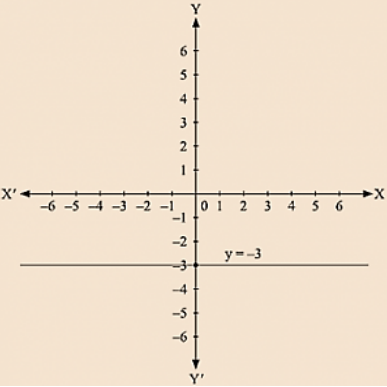(iii) we are given. y = 3

The representation of the solution on the number line. when given equation is treated as an equation in one variable.The representation of the solution on the Cartesian plane, it is a line parallel to x axis passing through the point (0, 3) is shown below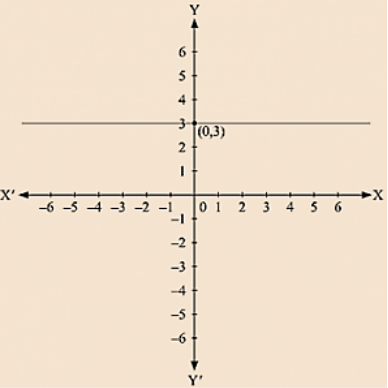(iv) We are given, 2x + 9 = 0

We get, 2x = - 9

The representation of the solution on the number line, when given equation is treated as an equation in one variable.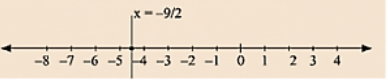The representation of the solution on the Cartesian plane, it is a line parallel to y axis passing through the point (-9/2, 0) is shown below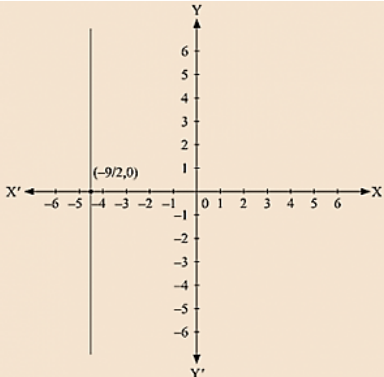(v) We are given, 3x - 5 = 0

We get, 3x = 5

The representation of the solution on the number line, when given equation is treated as an equation in one variable.The representation of the solution on the Cartesian plane, it is a line parallel to y axis passing through-the point (5, 0) is shown below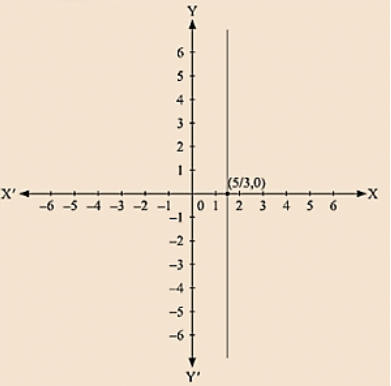Question: 2

Give the geometrical representation of 2x + 13 = 0 as an equation in

(i) one variable (ii) two variables

Solution:

We are given,

2x + 13 = 0

We get,

2x = -13

x = -13/2

The representation of the solution on the number line, when given equation is treated as an equation in one variable.The representation of the solution on the Cartesian plane, it is a line parallel to y axis passing through the point (-13/2, 0) is shown below.

Question: 3

Solve the equation 3x + 2 = x - 8, and represent the solution on

(i) the number line

(ii) the Cartesian plane.

Solution:

We are given,

3x + 2 = x - 8

we get,

3x - x = - 8 - 2

2x = - 10

x = - 5

The representation of the solution on the number line, when given equation is treated as an equation in one variable.The representation of the solution on the Cartesian plane, it is a line parallel to y axis passing through the point (- 5, 0) is shown below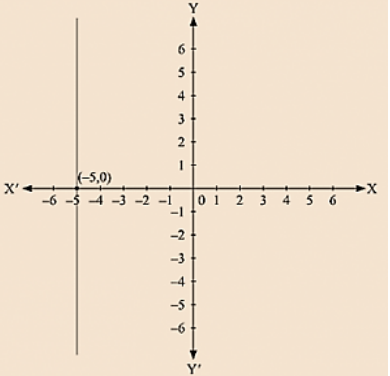Question: 4

Write the equation of the line that is parallel to x-axis and passing through the points

(i) (0, 3)

(ii) (0, - 4)

(iii) (2, – 5)

(iv) (3, 4)

Solution:

(i) We are given the co-ordinates of the Cartesian plane at (0, 3).

For the equation of the line parallel to x axis, we assume the equation as a one variable equation

independent of x containing y equal to 3.

We get the equation as y = 3

(ii) We are given the co-ordinates of the Cartesian plane at (0,- 4).

For the equation of the line parallel to x axis, we assume the equation as a one variable equation

Independent of x containing y equal to - 4.

We get the equation as y = - 4

(iii) We are given the co-ordinates of the Cartesian plane at (2, – 5).

For the equation of the line parallel to x axis, we assume the equation as a one variable equation

independent of x containing y equal to - 5.

We get the equation as y = - 5

(iv) We are given the co-ordinates of the Cartesian plane at (3, 4).

For the equation of the line parallel to x axis, we assume the equation as a one variable equation

independent of x containing y equal to 4.

We get the equation as

y = 4

Question: 5

Write the equation of the line that is parallel to y-axis and passing through the Points

(i) (4, 0)

(ii) (- 2, 0)

(iii) (3, 5)

(iv) (- 4, - 3)

Solution:

(i) We are given the coordinates of the Cartesian plane at (4, 0)

For the equation of the line parallel to y axis, we assume the equation as a one variable equation

independent of y containing x equal to 4

We get the equation as y = 3

(ii) We are given the coordinates of the Cartesian plane at (-2, 0)

For the equation of the line parallel to y axis, we assume the equation as a one variable equation

independent of y containing x equal to - 2

We get the equation as y = - 4

(iii) We are given the coordinates of the Cartesian plane at (3, 5)

For the equation of the line parallel to y axis, we assume the equation as a one variable equation

independent of y containing x equal to 3

We get the equation as y = -5

(iv) We are given the coordinates of the Cartesian plane at (- 4, -3)

For the equation of the line parallel to y axis, we assume the equation as a one variable equation

independent of y containing x equal to - 4

We get the equation as y = 4
```### Course Features

• 728 Video Lectures
• Revision Notes
• Previous Year Papers
• Mind Map
• Study Planner
• NCERT Solutions
• Discussion Forum
• Test paper with Video Solution